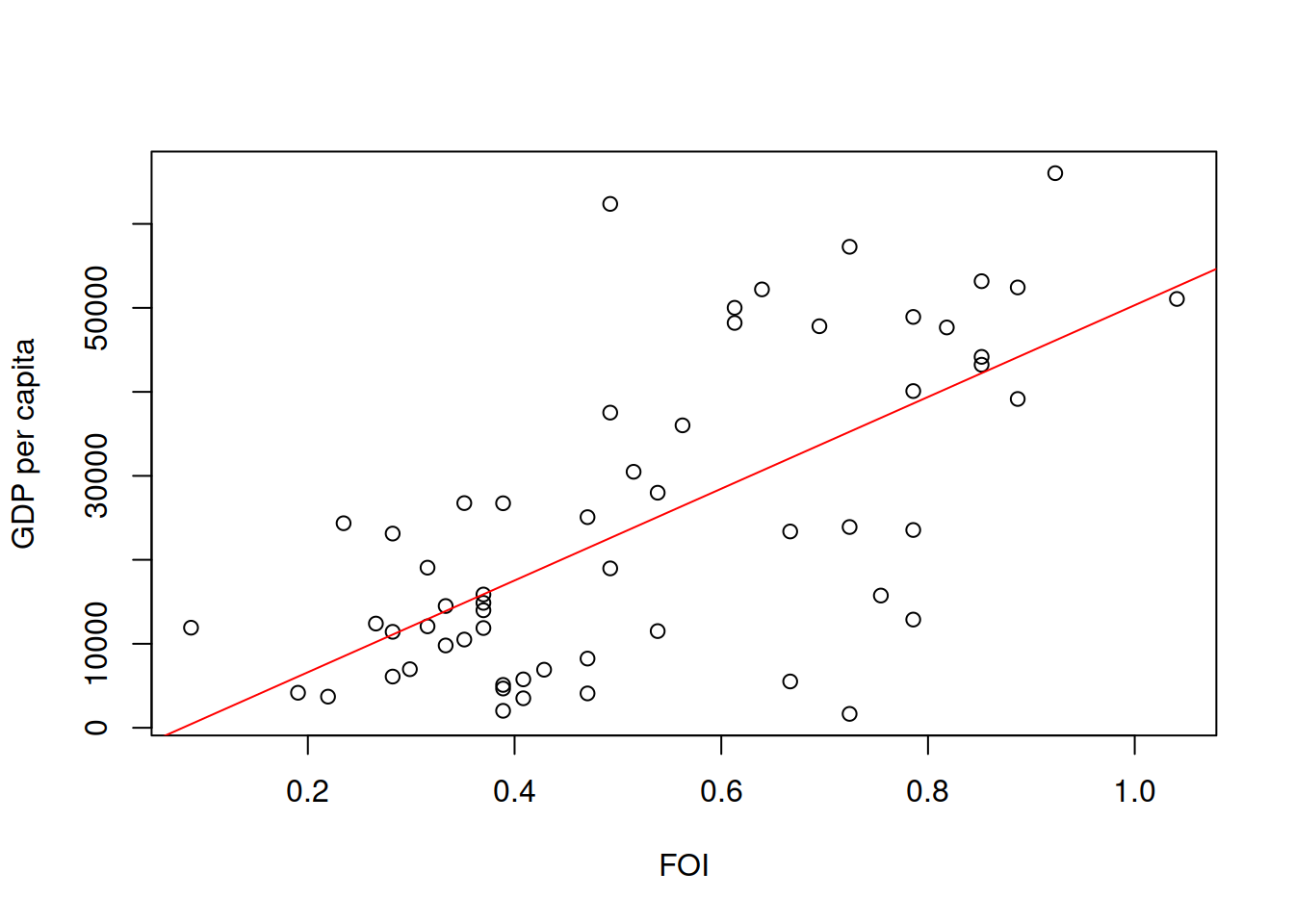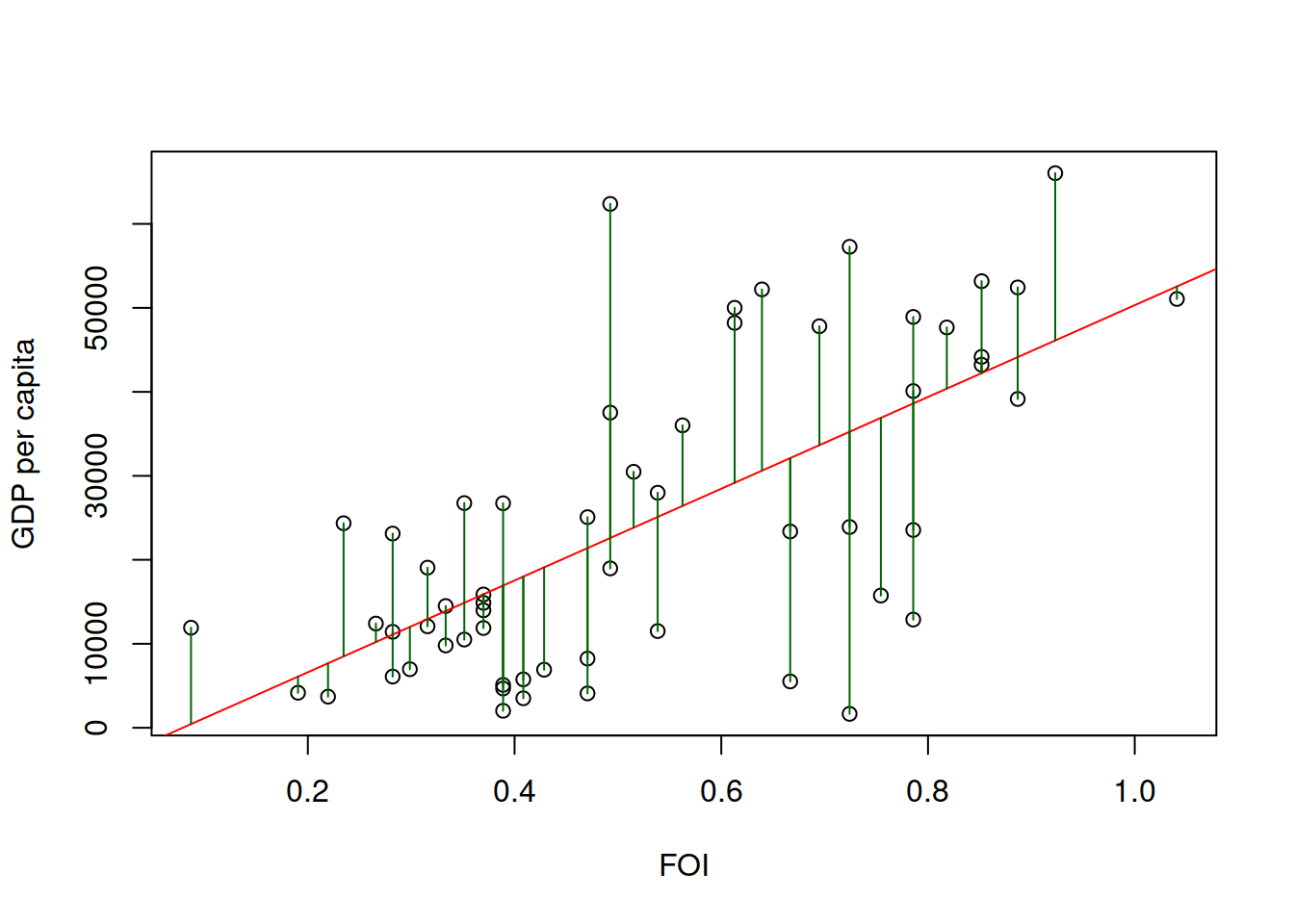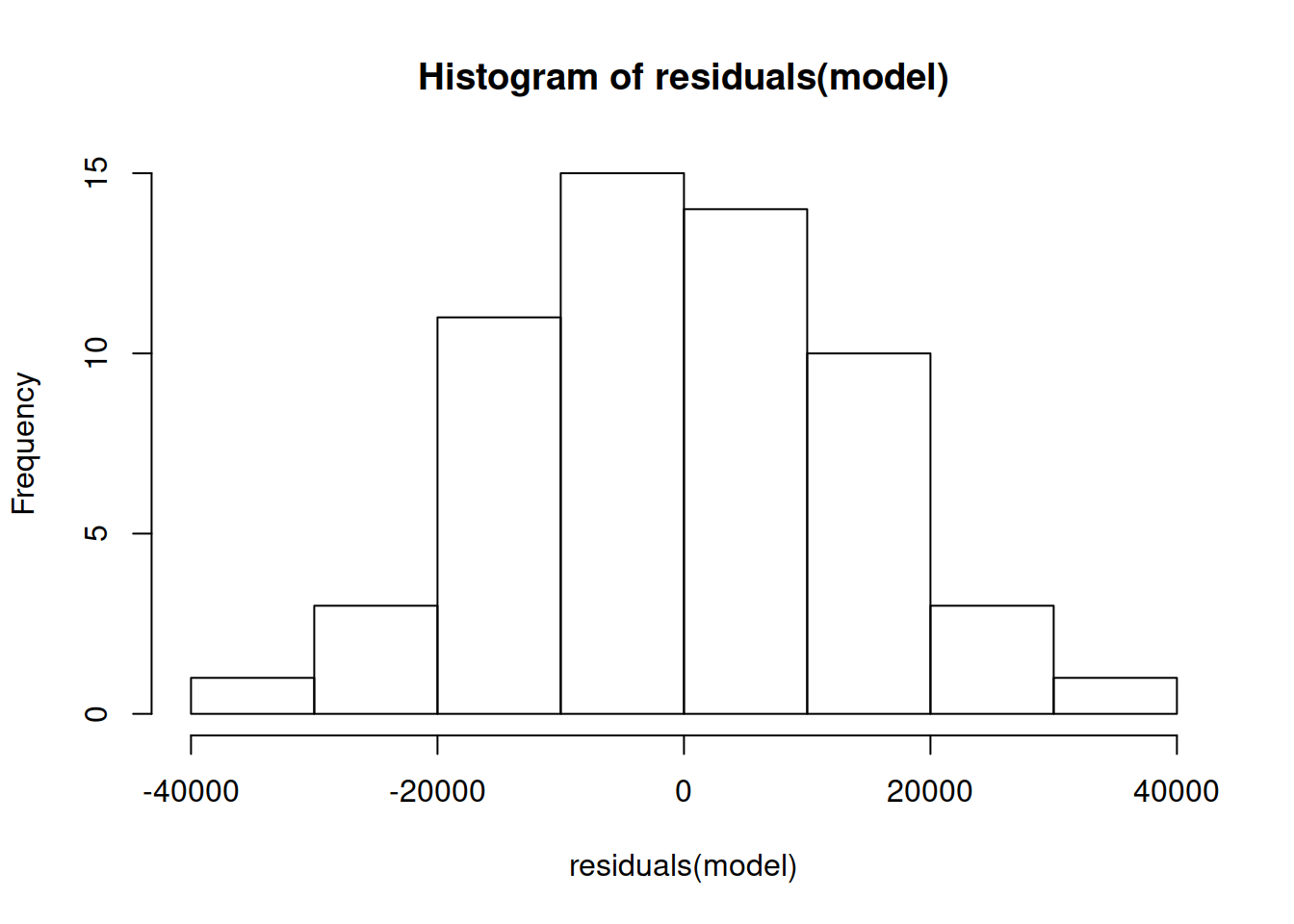### Regression analysis

Regression models formalize an equation in which one numeric variable $$Y$$ is formulated as a function of other variables $$X_1$$, $$X_2$$, $$X_3$$, etc:
$$Y = f(X_1,X_2,X_3...) + \epsilon$$

$$\epsilon$$ is called the residual, which is an error term in case the function does not fit perfectly $$Y$$.

In this tutorial we will learn about linear regression, which are regression models in which the function $$f()$$ is a linear combination of variables. More precisely:

$$Y = a + b_1 X_1 + b_2 X_2 + b_3 X_3 ... + \epsilon$$

• $$Y$$ is called the dependent variable
• $$X_1$$, $$X_2$$, $$X_3$$, etc are called independent variables
• $$a$$ is the intercept, which measures the expected value of $$Y$$ that does not depend on the dependent variables
• $$b_1$$, $$b_2$$, $$b_3$$, etc are called the slopes or the coefficients of the independent variables. They measure how much $$Y$$ grows with the corresponding variable. These coefficients are measure in the units of $$Y$$ divided by the units of $$X$$
• $$\epsilon$$ are the residuals, as in the equation before

For example, when we studied how GDP per capita depended on the FOI, we have a case where $$Y$$ is GDP and there is one independent variable $$X$$, the FOI. Here you see a scatter plot of GDP vs FOI with a line that shows a regression result:### Regression residuals

Residuals ($$\epsilon$$) are the differences in between the empirical values $$Y_i$$ and their fitted values $$\hat Y_i$$. In the following plot you see them for the case of GDP and FOI as vertical green lines:Linear regression analyses might have some assumptions regarding residuals. For example, the standard assumptions in many research projects is that residuals have zero mean, are normally distributed with some standard deviation ($$\epsilon \sim N(0,\sigma)$$, and that are uncorrelated with both $$X$$ and $$Y$$. At the end of this tutorial you have ways to inspect if these assumptions are met.

### Ordinary Least Squares (OLS)

Fitting a regression model is the task of finding the values of the coefficients ($$a$$, $$b_1$$, $$b_2$$, etc) in a way that reduce a way to aggregate the residuals of the model. One approach is called Residual Sum of Squares (RSS), which aggregates residuals as:
$$RSS = \sum_i (\hat Y_i - Y_i)^2$$

The Ordinary Least Squares method (OLS) looks for the values of coefficients that minimize the RSS. This way, you can think about the OLS result as the line that minimizes the sum of squared lengths of the vertical lines in the figure above.

### Regression in R

The lm() function in R fits a linear regression model with OLS. You have to specify the formula of your regression model. For the case of one independent variable, a formula reads like this:

DependentVariable ∼ IndependentVariable

If you print the result of lm(), you will see the best fitting values for the coefficients (intercept and slope):

model <- lm(GDP~FOI, df)
print(model)
##
## Call:
## lm(formula = GDP ~ FOI, data = df)
##
## Coefficients:
## (Intercept)          FOI
##       -4309        54631

You can access the estimated values of the coefficients like this:

model$coefficients ## (Intercept) FOI ## -4309.223 54631.170 Remember that the unit of the slope coefficient is measured in the units of $$Y$$ divided by the units of $$X$$. Since the FOI is a fraction, the slope here is telling us that an increase of one in the scale of FOI means an increase of $$54631$$ USD of GDP per capita. To see the regression result over a scatter plot as we did above, you can use the abline() function. To do so, set the first parameter of abline() as the intercept of the model and the second one as the slope: plot(df$FOI, df$GDP, xlab="FOI", ylab="GDP per capita") abline(model$coefficients, model$coefficients, col="red")You can access the residuals of the model with the residuals() function: head(residuals(model)) ## 1 2 3 4 5 6 ## -13594.855 -13159.734 -15065.169 7279.355 10946.910 -1021.044 and the estimated values $$\hat Y_i$$ with the predict() function. This function will apply the equation of our model $$a + b X$$ with the estimated values of the coefficients to the FOI values to predict the GDP values. The result is one estimate of $$Y$$ per row in the data: head(predict(model)) ## 1 2 3 4 5 6 ## 25107.56 21399.56 38615.27 40389.01 42228.44 15896.83 ### Goodness of fit After fitting the model, you should ask yourself how good are the predictions of the model or what is the quality of the fit. A way to measure this is to calculate the proportion of variance of the dependent variable ($$V[Y]$$) that is explained by the model. We can do this by comparing the variance of residuals ($$V[\epsilon]$$) to the variance of $$Y$$. If the variance of residuals is very small in comparison, we have a good fit. This is captured by the coefficient of determination, also known as $$R^2$$: $$R^2 = 1 − \frac{V[\epsilon]}{V[Y]}$$ For our model example: var(df$GDP)
##  336971949
var(residuals(model))
##  187606324
1-var(residuals(model))/var(df$GDP) ##  0.4432583 The function summary, among other things, calculates the $$R^2$$ of the model: summary(model) ## ## Call: ## lm(formula = GDP ~ FOI, data = df) ## ## Residuals: ## Min 1Q Median 3Q Max ## -33595 -10693 -446 9746 39780 ## ## Coefficients: ## Estimate Std. Error t value Pr(>|t|) ## (Intercept) -4309 4675 -0.922 0.361 ## FOI 54631 8182 6.677 1.18e-08 *** ## --- ## Signif. codes: 0 '***' 0.001 '**' 0.01 '*' 0.05 '.' 0.1 ' ' 1 ## ## Residual standard error: 13820 on 56 degrees of freedom ## Multiple R-squared: 0.4433, Adjusted R-squared: 0.4333 ## F-statistic: 44.59 on 1 and 56 DF, p-value: 1.18e-08 summary(model)$r.squared
##  0.4432583

The table shows you the estimates of the coefficients, in this case there is an intercept and a coefficient for FOI. The last column of the table shows what is called a p-value, if you want to learn more about that, check the permutation tests tutorial. The $$R^2$$ of 0.44 we get means that we can explain 44% of the variance of the GPD per capita with the FOI values of the countries alone.

The coefficient of determination is called $$R^2$$ because it is a way to measure the correlation coefficient between the true values of the dependent variable $$Y$$ and the estimated ones $$\hat Y$$. You can verify this in R:

summary(model)$r.squared ##  0.4432583 cor(df$GDP, predict(model))^2
##  0.4432583

You can specify models with more than one independent variable by using “+” in the formula. For example, for three independent variables:

DependentVariable ∼ IndependentVariable1 + IndependentVariable2 + IndependentVariable3

If we wanted to fit a model of GDP as a linear combination of the FOI and the internet penetration in countries, we can do it as follows:

model2 <- lm(GDP~FOI+IT.NET.USER.ZS, df)

And you can see the quality of the result like this:

summary(model2)
##
## Call:
## lm(formula = GDP ~ FOI + IT.NET.USER.ZS, data = df)
##
## Residuals:
##      Min       1Q   Median       3Q      Max
## -17051.4  -4697.5   -224.4   3577.9  19619.5
##
## Coefficients:
##                 Estimate Std. Error t value Pr(>|t|)
## (Intercept)    -16154.30    2951.46  -5.473 1.12e-06 ***
## FOI             20528.83    5776.48   3.554 0.000788 ***
## IT.NET.USER.ZS    539.85      51.55  10.473 1.04e-14 ***
## ---
## Signif. codes:  0 '***' 0.001 '**' 0.01 '*' 0.05 '.' 0.1 ' ' 1
##
## Residual standard error: 8058 on 55 degrees of freedom
## Multiple R-squared:  0.8141, Adjusted R-squared:  0.8073
## F-statistic: 120.4 on 2 and 55 DF,  p-value: < 2.2e-16

This $$R^2$$ of 0.81 is much higher than for the case without Internet penetration. The quality of the model has improved a lot, as Internet Penetration is also an important predictor of GDP besides what the FOI explains.

### Regression diagnostics

Common assumptions of regression models relate to the distribution of residuals and their correlation with other variables. Later in this course you will learn methods that do not have many assumptions like these, but in case you use any model, read the documentation to learn its assumptions and then see if they are met with R.

For example, we want the model to be unbiased in the sense that it cannot be easily improved by changing the value of the coefficients. You can verify this by checking that the mean value of residuals is very close to zero:

mean(residuals(model))
##  1.011268e-12

A common assumption is that residuals are normally distributed, which is a necessary condition for the additional information in the summary tables to be correct. You can see the distribution of residuals with a histogram:

hist(residuals(model))The example above is close to a normal distribution, as it is symmetric, has a mode in the center, and the characteristic bell curve of a normal distribution.

Other assumptions are that residuals are uncorrelated with predicted values:

cor(residuals(model), predict(model))
##  -1.058338e-17

and that the variance of residuals is constant across the predicted value range. You can have an idea of that by looking at a scatter plot of residuals and predicted values:

plot(predict(model), residuals(model))